## Resource of the Week: Pairs that make 20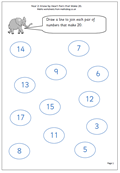This week it is a page from our year 2 worksheets which looks at pairs of numbers which make 20. Before doing this children should be confident with, and know by heart, all pairs of numbers which make 10. Once they know these, pairs that make 20 becomes easy; just one of each pair is ten more e.g. 6 and 4: 16 and 4. Being able to work quickly with mental arithmetic is one of the keys to success in school as so much of the maths lesson today is carried out orally and children gain or lose confidence very quickly when answering in front of a whole class.

Know  pairs that make 20 (pg 1)

## Year 1 Maths worksheet: Handling data and sorting shapes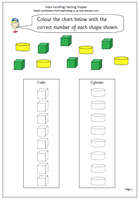One of the earliest stages of data handling is to be able to sort and a lot of practical work can be done, such as tidying up a drawer of pens, pencils, paper clips etc.

The next stage is to be able to record the results and here we have a simple page where the two sets of shapes can be sorted and recorded on the columns.

The outlines of the cylinders and cubes are provided and it is just a matter of counting the number of each shape and recording by colouring the correct number on the columns.

This page can be found in our Handling Data section for Year 1.

Sorting shapes 1

## Year 6 Maths: Fractions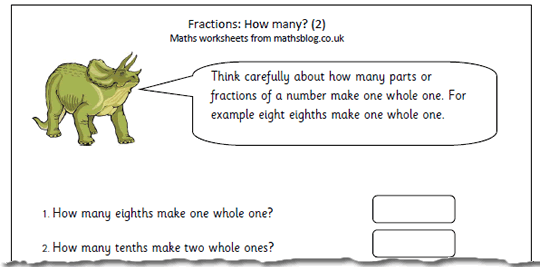This is the second page where we look at questions such as:

‘How many sixths make two and a half?’

Children need to know that there are six sixths in one whole one and also need to understand equivalent fractions and that a half is equivalent to three sixths.

Many children fail to grasp this in primary school, probably because they have never really got to grips with equivalent fractions and it might be necessary to go back and practice this first before attempting these questions.

How many fractions make…(pg 2)

## Coming soon: Fractions, handling data and multiplesA real mixed bag of pages coming up next week.

We will post the  second page where we look at questions such as:

‘How many sixths make two and a half?’

Children need to know that there are six sixths in one whole one and also need to understand equivalent fractions and that a half is equivalent to three sixths.

An important early stage in Handling Data is to be able to record the results and we will be publishing a simple page where  two sets of shapes can be sorted and recorded on the columns.

The outlines of the cylinders and cubes are provided and it is just a matter of counting the number of each shape and recording by colouring the correct number on the columns.

Also coming soon is another in our series of fun games to play, suitable for year 3 children who have got a good grasp of the three times table.

## Year 5 maths worksheet: Percentages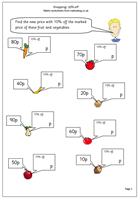A straightforward introduction to finding 10% off multiples of 10p up to a pound. This can be done in two stages:

Firstly: find 10% of the total. As these are all multiples of ten this is straightforward – just divide the amount by 10.

eg 10% of 50p is 5p

Secondly: subtract from the original amount.

50p subtract 5p is 45p.

However, if you have some really nifty mathematicians they might spot that if you multiply the 5(tens) by 9 you get 450p and divide by 10 to get 45p (or simply multiply 5 by 9). This is a case of finding 90% rather than finding 10% and subtracting from 100%. Tricky, eh?

10 percent off

## Resource of the Week: Decimals that make 10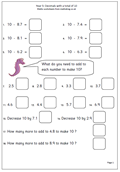Here we have mental arithmetic maths worksheet on decimals, most suitable for Year 5. This page looks at pairs of decimals that make 10. Once again there are several ways of tackling these questions.

One way is to add on from the smaller number, firstly, the tenths to make the next whole number, and then the units to make 10.

eg 10 – 6.7

Count on from 6.7 to 7 which is 0.3

Then count on from 7 to 10 which is 3

Answer 3 + 0.3 = 3.3

Another way is to count on the units first and then the tenths, but beware the answer which counts on 4 from 6 to make 10 and than counts on 0.3 as this will result in an answer which is one too many: a common slip-up.

Another way of doing this is to ignore the decimal and think of the question as 100 – 67. Work it out mentally (which is 33) and then convert back to 3.3.

Decimals with a total of 10

## Year 4 maths worksheet: Division with remainders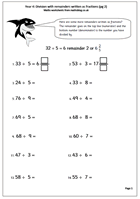Here is another page where the remainders from division are written as fractions. Children need a good knowledge of tables to work out the division ‘in their heads’ and this is probably best suited to Year 4, aged8/9+ years old.

The remainder goes on the top line (numerator) and the bottom number (denominator) is the number you have divided by.

This is a much neater finish to the question as the whole number is divided completely, with no messy remainders. Some of the fractions could be cancelled down to their simplest form, although this is not essential at this stage.

Division with fraction remainders (pg 2)

## Year 1 3D shape worksheet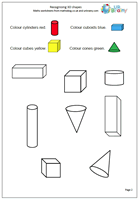The best way to learn about 3D shapes is to handle them but it is also important to recognise drawings of them on paper. Four shapes which children should be familiar with are the cube, cuboid, cylinder and cone. here we have a page which asks children to recognise these shapes and colour them accordingly.

Thanks to urbrainy.com for letting me use this page from their huge collection of resources for Early Years.

3d shape (pg 1)

## Coming soon: 3D shape, division with remainders and percentagesA real cross section of resources coming up next week. To start with we have a 3D shape page for year 1. The best way to learn about 3D shapes is to handle them but it is also important to recognise drawings of them on paper. Four shapes which children should be familiar with are the cube, cuboid, cylinder and cone.

Next we have another page where the remainders from division are written as fractions. Children need a good knowledge of tables to work out the division ‘in their heads’ and this is probably best suited to Year 4, aged8/9+ years old.

Also next week there is a straightforward introduction to finding 10% off multiples of 10p up to a pound. This is ideal for year 5 children with a good understanding of multiplication and division by 10.

## Calculator game: Multiples of 2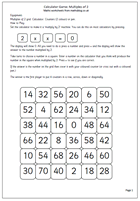This is a harder version of the 2x table calculator game, published earlier in that the grid has larger multiples of 2. All you need is the grid, some counters or cubes and a calculator. Set the calculator up as a 2x table answer machine by keying in 2, x, x, =, 0 and then all you need to do is press a number and the equals sign and it will show the answer to the number multiplied by 2. Just type in the next number, press equals and so on…

Once again, the aim of the game is to make a line of four in a row in any direction.

Multiples of 2